Reach Us+44-1522-440391
On Poincare Polynomials of Hyperbolic Lie Algebras | OMICS International
Journal of Generalized Lie Theory and Applications
All submissions of the EM system will be redirected to Online Manuscript Submission System. Authors are requested to submit articles directly to Online Manuscript Submission System of respective journal.

# On Poincare Polynomials of Hyperbolic Lie Algebras

Gungormez M* and Karadayi HR

Department of Physics, Faculty of Sciences and Letters, Istanbul Technical University, Maslak, 34469 Istanbul, Turkey

*Corresponding Author:
Gungormez M
Department of Physics
Faculty of Sciences and Letters
Istanbul Technical University
Maslak, 34469 Istanbul, Turkey
Tel: +90 212 2853220
Fax: +90 212 2856386
E-mail: [email protected]

Received Date: January 11, 2017; Accepted Date: February 17, 2017; Published Date: February 27, 2017

Citation: Gungormez M, Karadayi HR (2017) On Poincare Polynomials of Hyperbolic Lie Algebras. J Generalized Lie Theory Appl 11: 255. doi:10.4172/1736-4337.1000255

Copyright: © 2017 Gungormez M, et al. This is an open-access article distributed under the terms of the Creative Commons Attribution License, which permits unrestricted use, distribution, and reproduction in any medium, provided the original author and source are credited.

Visit for more related articles at Journal of Generalized Lie Theory and Applications

#### Abstract

We have general frameworks to obtain Poincare polynomials for Finite and also Affine types of Kac-Moody Lie algebras. Very little is known however beyond Affine ones, though we have a constructive theorem which can be applied both for finite and infinite cases. One can conclusively said that theorem gives the Poincare polynomial P(G) of a Kac-Moody Lie algebra G in the product form P(G)=P(g) R where g is a precisely chosen sub-algebra of G and R is a rational function. Not in the way which theorem says but, at least for 48 hyperbolic Lie algebras considered in this work, we have shown that there is another way of choosing a sub-algebra in such a way that R appears to be the inverse of a finite polynomial. It is clear that a rational function or its inverse can not be expressed in the form of a finite polynomial.

Our method is based on numerical calculations and results are given for each and every one of 48 Hyperbolic Lie algebras.

In an illustrative example however, we will give how above-mentioned theorem gives us rational functions in which case we find a finite polynomial for which theorem fails to obtain.

#### Introduction

A characteristic fact about Poincare polynomial P(GN) of a Kac- Moody Lie algebra  GN of rank N is that the term of an order s gives the number of its Weyl group elements which are composed out of the products of s number of simple Weyl reflections corresponding to simple roots of GN . For finite Lie algebras, Poincare polynomials are known  in the following form: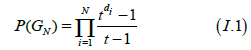Where di’s are the degrees of N basic invariants of GN and t is always assumed to be an indeterminate. For an affine Kac-Moody Lie algebra GN originated from a generic finite Lie algebra Gn where in general N>=n, Bott theorem  states that its Poincare polynomial has the following product form: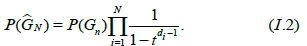Beyond Affine Kac-Moody Lie algebras, we only know a theorem p.123 of ref.  which shows out a specific way to obtain Poincare polynomial in any case, finite or infinite. It is this theorem which in fact can be applied also in obtaining of (I.1) and also (I.2). Theorem says that the Poincare polynomial P(GN) of a Kac-Moody Lie algebra GN has the product form:

P(Gn)=P(gn)R (I.3)

where P(gn) is also the Poincare polynomial of a sub-algebra gn⊂ GN with N>n. It is trivial to see that theorem requires gn should be contained inside the Dynkin diagram of GN. Our concern in this work is the proposition of this theorem which says that R is a rational function. For a Hyperbolic Lie algebra Hi, our observation on the other hand is that its Poincare polynomial comes in the form:

P(Hi)=P(G) Q (G) (I.4)

where G is a properly chosen finite Lie Algebra and Qi(G) is a polynomial of some finite degree in indeterminate t. Due to the fact that a rational function or its inverse can only be represented by a polynomial of infinite order, it is clear that (I.3) and (I.4) say different and hence our reasoning in this work is free of what the theorem says beyond Affine Kac-Moody Lie algebras.

It is known that there are a finite number of Hyperbolic Lie algebras . In the next section, we show how we obtain Poincare polynomials for 48 one of them and hence the generalized form (I.4). There are two points to mention here;

(1) Degrees of polynomials Qi(G) come in two different values D or D-1 where D is the number of positive roots of the chosen finite Lie algebra G. (2) For the same Hi, there could be several equivalent ways to specify the finite Lie algebra G so the explicit form of denominator polynomials Qi(G) depends on this choice. What is important here is however that, on the contrary to above-mentioned theorem, there is no any requirement that G should be contained inside the Dynkin diagram of Hi.

The following 3 examples will be instructive in all these points and also reflect the basics of our calculational algorithm. In general terms, let W(GN) be the Weyl group of GN and σi’s be its elements corresponding to simple roots σi’s where i=1,…,N. In the following, we assume that reduced forms of elements of a Weyl group can be expressed by the following notation: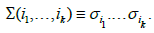Let S={α1,…,α6} be the set of simple roots of H48 for which we use the following. Dynkin diagram for the first 2 examples: (Figure 1), =6 cm.

Among several possible choices, our 2 examples lead us respectively to sub-algebras A4 and D5 of H48, in view of the following choices of 2 subsets of S: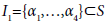(1)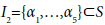(2)

At this point, one must notice that there could be no choice for a subset which allows us to get a Lie sub-algebra which is not contained inside the Dynkin diagram of H48. It is now seen for the first case that the above-mentioned theorem leads us to a Poincare polynomial:

P(H48)=P(A4) R1 (I.5)

where P(A4) is the Poincare polynomial of A4 Lie algebra with the Dynkin diagram (Figure 2), and R1 is a rational function which we calculate here, explicitly. In favor of (I.1), we know that: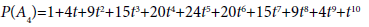and corresponding 120 elements of W(H48) form a subset W(A4)⊂W(H48). Among infinite number of elements of W(H48), there are only 120 elements originate only from the set I1⊂S of example (1).

In (I.5), on the other hand, R1 originates from the subset R1(H48)⊂W(H48) for which any element w∈W(H48) can be expressed by the factorized form:

w=uv, u∈W(A4), v∈R1(H48) (I.6)

in such a way that:

l(w))=l(u))+l(v) (I.7)

where l is the length function of H48. To exemplify our algorithm, we give in the following the elements of R1(H48) up to 6th order: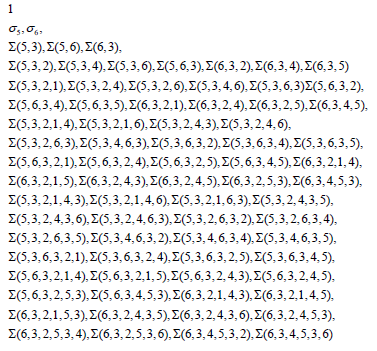The reader could verify order by order that the number of these elements do match with the first 6 terms in the infinite polynomial expansion of the following rational function: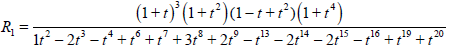Our algorithm however allows us to investigate the existence of (I.5) at any order. To this end, let us define: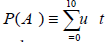and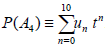Since R1 is a rational function, it could be represented also by a polynomial of infinite order: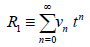The reader could also verify now that at any order M=0,…,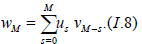In case of example (2), one finds the following Dynkin diagram (Figure 3), which gives us:

P(H48)=P(D5)R2 (I.9)

where P(D5) is the Poincare polynomial of D5 Lie algebra. As in the first example, R2 is to be calculated in the form of the following rational polynomial :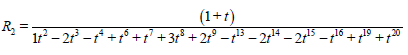and the similar of (I.8) is seem to be valid.

For our last example, the Dynkin diagram of H48 should be defined, not in the way defined above but as in the following: (Figure 4) in such a way that the choice: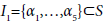(3)

gives us an infinite sub-algebra which is in fact the affine Lie algebra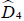with the following Dynkin diagram: (Figure 5).

This time, similar of (I.5) and (I.9) is obtained in the form: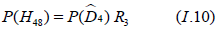for which one has: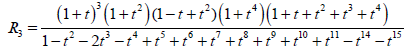In accordance with (I.2), with the notice that: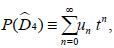one sees the similar of (I.8) is also valid here.

Let us conclude this section by giving the main motivation behind this work against the well known existence of the above-mentioned factorization theorem for Poincare polynomials, in general. As the theorem said, in all examples corresponding polynomials R1,R2 and also R3 are rational functions. In the next section, we show however that, for Poincare polynomials of 48 Hyperbolic Lie algebras, there is another factorization (I.4). We know from above discussion that theorem could not give a way for obtaining such a factorization.

#### Calculation of Hyperbolic Poincare Polynomials

We follow the notation of Kac-Moody-Wakimoto  for hyperbolic Lie algebras. To explain formal structure of our calculations, we follow the example H48 from Appendix. Its simple roots αi and fundamental dominant weights λi are given by equations: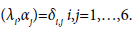where κ(,) is the symmetric scalar product which we know to be exist, on H48 weight lattice, by its Cartan matrix A.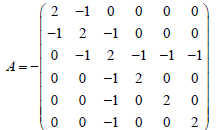so we have: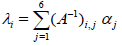As in above, let GN be a chosen Kac-Moody Lie algebra, W(GN) be its Weyl group and ρ its Weyl vector. For any Σ∈W(GN), let us now consider: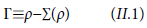which is by definition an element of the positive root lattice of GN. We know that Γ is unique in the sense that Γ≡ρ−Σ(ρ) is different from Γ′≡ρ−Σ′(ρ) for any two different Σ, Σ′∈W(GN). This could be easily understood due to definition of Weyl vector which is in fact a strictly dominant weight. This is sufficient to suggest our simple method to calculate the number of Weyl group elements which are expressed in terms of the same number of simple Weyl reflections σi which are defined by: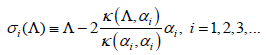for any element Λ of weight lattice. Let us now consider k-tuple products: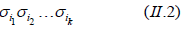which can not be reduced into products consisting less than k-number of simple Weyl reflections, that is reduced elements. Out of all these reduced elements as in (II.2), we define a class Wk⊂W(GN). The elements of any class Wk are to be determined uniquely by their actions on the Weyl vector which are different from each other. We use a definitive algorithm to choose the ones among the equivalents so, as is emphasized in the 3 examples given above, our way of choosing the Weyl group elements originating from the subsets (1), (2) and (3) is based on this algorithm. The aim of this work doesn’t need to show this algorithm here, in an explicit way.

Now we can formally state that a Weyl group is the formal sum of its classes Wk. One should note that the number of elements of Wk is always finite though the number of classes is finite for finite and infinite for infinite Kac-Moody Lie algebras.

Looking back to H48, we give some of its classes in the following: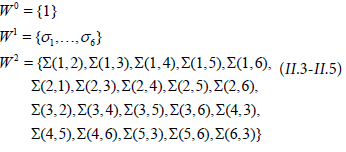By setting |Wk| to be the order of the set Wk, one has the polynomial: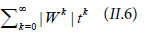which is nothing but the Poincare polynomial of H48. By explicit calculation up to 26th order, we obtained the following result: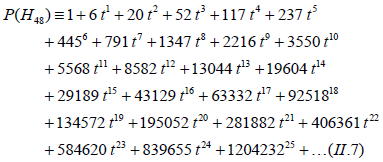One sees that (II.7) is enough to conclude that: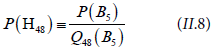Where,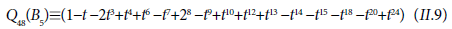and P(B5) is as given in (I.1) for B5 Lie algebra. As is emphasized in the first section, note here that the number of positive roots of B5 is equal to D=25 and hence Q48 (B5) is a polynomial of order D-1=24.

The results of our calculations for 48 Hyperbolic Poincare polynomials will be given in the following. Corresponding Dynkin diagrams will also be given in Appendix.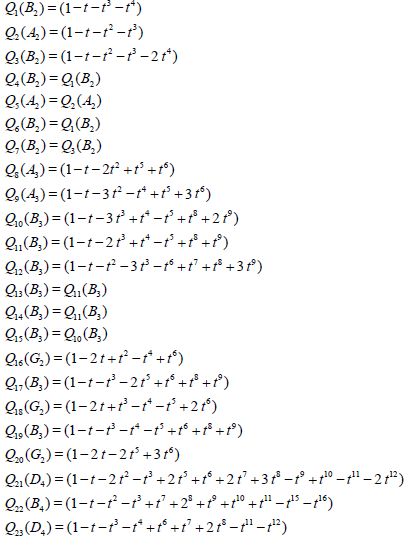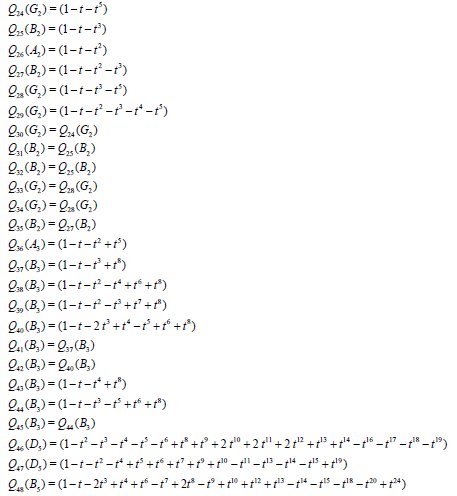#### Conclusion

Let us conclude with the main idea of this work by the aid of a beautiful example. One could say, for instance, that Bott theorem and also the factorization theorem given above say the same thing. Although this theorem proves useful as a calculational tool, Bott theorem gives us a general framework to apply for affine Lie algebras due to the fact that explicit calculation of a rational function is in effect quite hard if it is not impossible. We note again that a rational function can be expressed only in the form of a polynomial of infinite order.

In the lack of such a general framework for Lie algebras beyond affine ones, we also use an algorithm for explicit calculations. Against the mentioned theorem, explicit calculations are possible here due to the fact that in our formalism we only deal with polynomials of some finite degree.

In a future publication, we also consider the case beyond Hyperbolic Lie algebras, Kac-Moody Lie algebras of indefinite type (See Appendix).

References

Select your language of interest to view the total content in your interested language

### Article Usage

• Total views: 1011
• [From(publication date):
April-2017 - Aug 25, 2019]
• Breakdown by view type
• HTML page views : 923
• PDF downloads : 88

## Post your commentCan't read the image? click here to refresh
###### Peer Reviewed Journals

Make the best use of Scientific Research and information from our 700 + peer reviewed, Open Access Journals

International Conferences 2019-20

Meet Inspiring Speakers and Experts at our 3000+ Global Annual Meetings

Top